# 推荐算法之贝叶斯个性化排序 BPR

## 排序学习设计方法

### 单点法（Pointwise）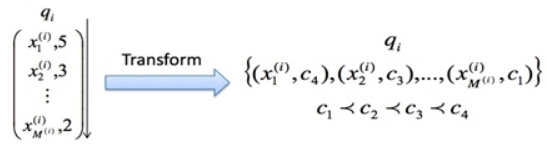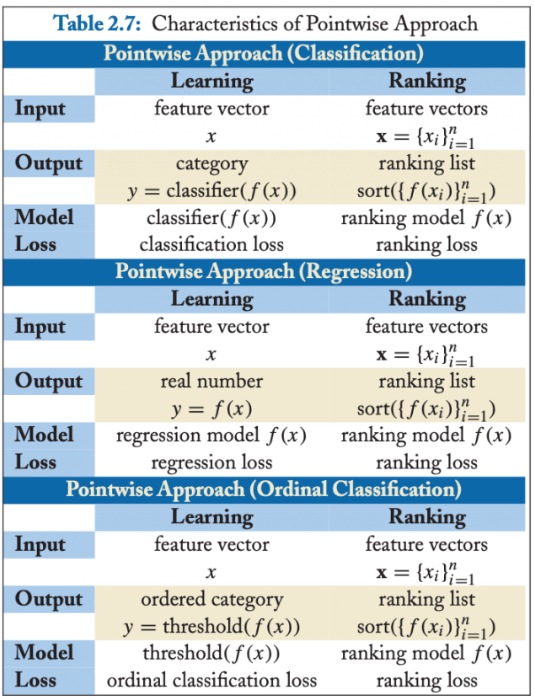• 分类（Classification）：输出空间包含的是无序类别，对每个查询-文档对的样本判断是否相关，可以是二分类的，如相关认为是正例，不相关认为是负例；也可以是类似 NDCG 那样的五级标注的多分类问题。分类模型通常会输出一个概率值，可根据概率值的排序作为排序最终结果。
• 回归（Regression）：输出空间包含的是真实值相关度得分，可通过回归来直接拟合相关度打分。
• 有序分类（Ordinal Classification）：有序分类也称有序回归（Ordinal Regression），输出空间一般包含的是有序类别，通常的做法是找到一个打分函数，然后用一系列阈值对得分进行分割，得到有序类别。

Pointwise 方法通过优化损失函数求解最优的参数，可以看到 Pointwise 方法非常简单，工程上也易实现，但是 Pointwise 也存在很多问题：

• Pointwise 只考虑单个文档同 query 的相关性，没有考虑文档间的关系，然而排序追求的是排序结果，并不要求精确打分，只要有相对打分即可；
• 通过分类只是把不同的文档做了一个简单的区分，同一个类别里的文档则无法深入区别，虽然我们可以根据预测的概率来区别，但实际上，这个概率只是准确度概率，并不是真正的排序靠前的预测概率；
• Pointwise 方法并没有考虑同一个 query 对应的文档间的内部依赖性。一方面，导致输入空间内的样本不是 IID 的，违反了 ML 的基本假设，另一方面，没有充分利用这种样本间的结构性。其次，当不同 query 对应不同数量的文档时，整体 loss 将容易被对应文档数量大的 query 组所支配，应该每组 query 都是等价的才合理。
• 很多时候，排序结果的 Top N 条的顺序重要性远比剩下全部顺序重要性要高，因为损失函数没有相对排序位置信息，这样会使损失函数可能无意的过多强调那些不重要的 docs，即那些排序在后面对用户体验影响小的 doc，所以对于位置靠前但是排序错误的文档应该加大惩罚。

### 配对法（Pairwise）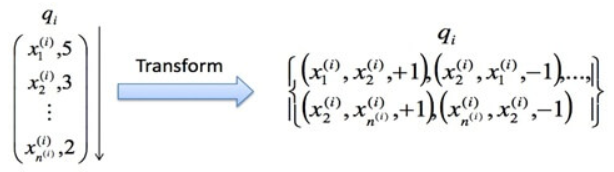• 我们可以针对某一个关键字得到一个完美的排序关系。在实际操作中，这个关系可以通过五级相关标签来获得，也可以通过其他信息获得，比如点击率等信息。然而，这个完美的排序关系并不是永远都存在的。试想在电子商务网站中，对于查询关键字 “哈利波特”，有的用户希望购买书籍，有的用户则希望购买含有哈利波特图案的 T 恤，显然，这里面就不存在一个完美排序。
• 我们寄希望能够学习文档之间的两两配对关系从而 “重构” 这个完美排序。然而，这也不是一个有 “保证” 的思路。用刚才的例子，希望学习两两关系 “B>C”、“B>A” 和 “C>A” 来重构完美排序 “B>C>A”。然而，实际中，这三个两两关系之间是独立的。特别是在预测的时候，即使模型能够正确判断 “B>C” 和 “C>A”，也不代表模型就一定能得到 “B>A”。注意，这里的关键是 “一定”，也就是模型有可能得到也有可能得不到。两两配对关系不能 “一定” 得到完美排序，这个结论其实就揭示了这种方法的不一致性。也就是说，我们并不能真正保证可以得到最优的排序。
• 我们能够构建样本来描述这样的两两相对的比较关系。一个相对比较简单的情况，认为文档之间的两两关系来自于文档特征（Feature）之间的差异。也就是说，可以利用样本之间特征的差值当做新的特征，从而学习到差值到相关性差异这样的一组对应关系。

Pairwise 最终的算分，分类和回归都可以实现，不过最常用的还是二元分类，如下图：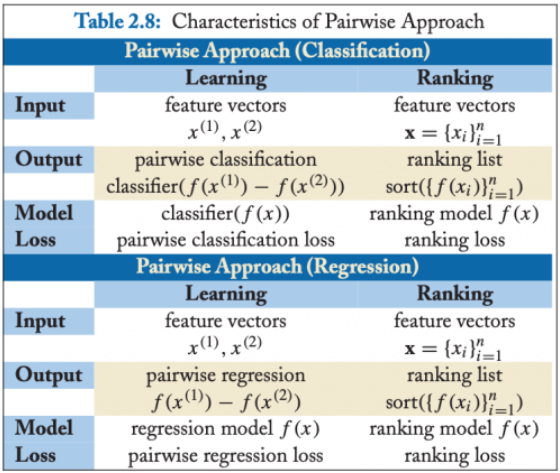Pairwise 方法通过考虑两两文档之间的相关对顺序来进行排序，相比 Pointwise 方法有明显改善。但 Pairwise 方法仍有如下问题：

• 使用的是两文档之间相关度的损失函数，而它和真正衡量排序效果的指标之间存在很大不同，甚至可能是负相关的，如可能出现 Pairwise Loss 越来越低，但 NDCG 分数也越来越低的现象。
• 只考虑了两个文档的先后顺序，且没有考虑文档在搜索列表中出现的位置，导致最终排序效果并不理想。
• 不同的查询，其相关文档数量差异很大，转换为文档对之后，有的查询可能有几百对文档，有的可能只有几十个，这样不加均一化地在一起学习，模型会优先考虑文档对数量多的查询，减少这些查询的 loss，最终对机器学习的效果评价造成困难。
• Pairwise 方法的训练样例是偏序文档对，它将对文档的排序转化为对不同文档与查询相关性大小关系的预测；因此，如果因某个文档相关性被预测错误，或文档对的两个文档相关性均被预测错误，则会影响与之关联的其它文档，进而引起连锁反应并影响最终排序结果。

### 列表法（Listwise）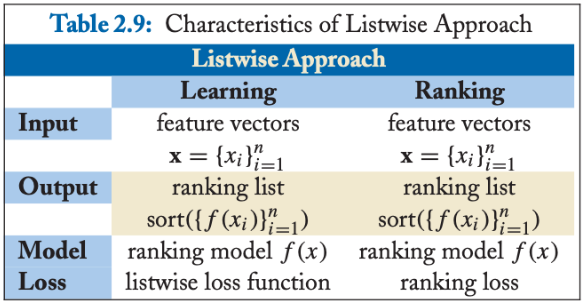1）Measure-specific，直接针对 NDCG 类的排序指标进行优化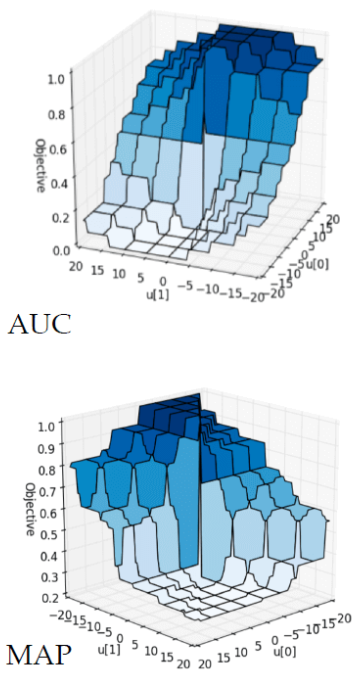• 既然直接优化有难度，那就找一个近似 NDCG 的另外一种指标。而这种替代的指标是 “连续” 和 “可微分” 的 。只要我们建立这个替代指标和 NDCG 之间的近似关系，那么就能够通过优化这个替代指标达到逼近优化 NDCG 的目的。这类的代表性算法的有 SoftRank 和 AppRank。
• 尝试从数学的形式上写出一个 NDCG 等指标的 “边界”（Bound），然后优化这个边界。比如，如果推导出一个上界，那就可以通过最小化这个上界来优化 NDCG。这类的代表性算法有 SVM-MAP 和 SVM-NDCG。
• 希望从优化算法上下手，看是否能够设计出复杂的优化算法来达到优化 NDCG 等指标的目的。对于这类算法来说，算法要求的目标函数可以是 “非连续” 和 “非可微分” 的。这类的代表性算法有 AdaRank 和 RankGP。

2）Non-measure specific，尝试重建最优顺序，衡量其中差异

3）列表法和配对法的中间解法

## BPR（贝叶斯个性化排序）简介

• 每个用户之间的偏好行为相互独立，即用户 u 在商品 i 和 j 之间的偏好和其他用户无关。
• 同一用户对不同物品的偏序相互独立，也就是用户 u 在商品 i 和 j 之间的偏好和其他的商品无关。

### 定义

U代表所有的用户user集合；I代表所有的物品item集合；S代表所有用户的隐式反馈，$S \subseteq U \times I$。如下图所示，只要用户对某个物品产生过行为，就标记为+, 所有+样本构成了S。那些未观察到的数据（即用户没有产生行为的数据）标记为?。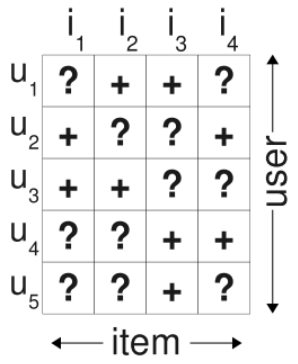$I_u^+=\{i \in I: (u,i) \in S\}$代表了用户u产生过行为的物品集合

$U_i^+=\{u \in U: (u,i) \in S\}$代表了对物品i产生过行为的用户集合

• 完整性：$\forall i,j \in I : i\neq j \Rightarrow i > _u j \cup j > _u i$
• 反对称性：$\forall i,j \in I : i > _u j \cap j > _u i \Rightarrow i = j$
• 传递性：$\forall i,j,k \in I : i > _u j \cap j > _u k \Rightarrow i > _u k$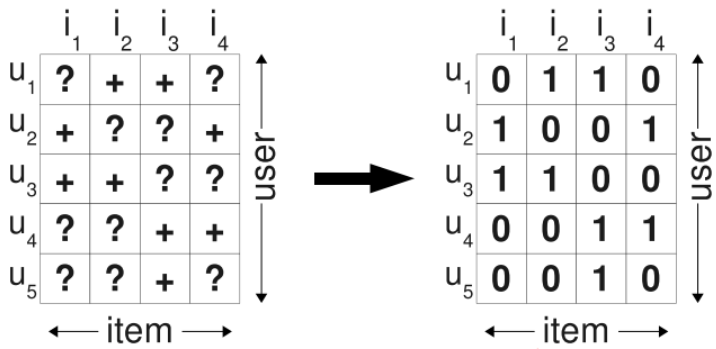BPR 的样本构建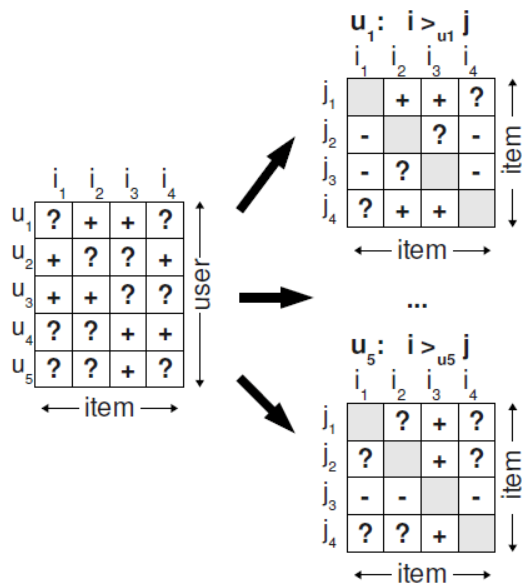## BPR（贝叶斯个性化排序）模型

BPR模型本质上是一种矩阵分解算法，所以其模型本质上是为了将用户物品矩阵分解成两个低维矩阵，再由两个低维矩阵相乘后得到完整的矩阵。对于用户集U和物品集I对应的U*I的预测排序矩阵，我们期望得到两个分解后的用户矩阵$W(\left |U \right |\times k)$ 和物品矩阵$H(\left |I \right |\times k)$，满足：

$$\bar{X} = WH^T$$

$$\bar{x} = w_u \cdot h_i = \sum_{f=1}^{k}w_{uf}h_{if}$$

### 算法运算思路

BPR 基于最大后验估计$P(W,H|>_u)$来求解模型参数W、H，这里我们用$\theta$来表示参数W和H，$>_u$代表用户u对应的所有商品的全序关系，则优化目标是$P(\theta | >_u )$。根据贝叶斯公式，我们有：

$$P(\theta | >_u ) = \frac{P(>_u | \theta)P(\theta)}{P(>_u)}$$

$$P(\theta | >_u ) \propto P(>_u | \theta)P(\theta)$$

$$\prod_{u\in U}P(>_u|\theta ) = \prod_{(u,i,j)\in (U\times I\times I)}P(i>_uj|\theta)^{\delta ((u,i,j)\in D)}(1-P(i>_uj|\theta))^{\delta ((u,i,j)\notin D)}$$

$$\delta (b) = \begin{cases} 1 & \text{ if b is true}\\ 0 & \text{ else } \end{cases}$$

$$\prod_{u \in U}P(>_u|\theta) = \prod_{(u,i,j) \in D}P(i>_uj|\theta)$$

$$P(i >_{u}j|\theta) =\sigma(\bar{x}_{uij}(\theta))$$

$$\bar{x}_{uij}(\theta) = \bar{x}_{ui}(\theta) – \bar{x}_{uj}(\theta)$$

$$\bar{x}_{uij} = \bar{x}_{ui} – \bar{x}_{uj}$$

$$\prod_{u \in U}P(>_u|\theta) = \prod_{(u,i,j) \in D}\sigma (\bar{x}_{ui}-\bar{x}_{uj})$$

$$P(\theta) \sim N(0,\lambda _\theta I)$$

$$\ln P(\theta) = \lambda \left \| \theta \right \|^2$$

$$\ln P(\theta |>_u) \propto \ln P(>_u |\theta)P(\theta) = \ln \prod_{(u,i,j)\in D}\sigma (\bar{x}_{ui}-\bar{x}_{uj}) + \ln P(\theta) = \sum_{(u,i,j) \in D}\ln \sigma (\bar{x}_{ui}-\bar{x}_{uj}) + \lambda \left \| \theta \right \|^2$$

### 算法代码实现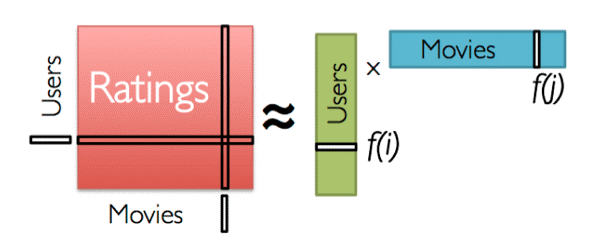import numpy as np
import pandas as pd
from collections import defaultdict
import random
import tensorflow as tf

def gen_test(user_ratings):
"""
对每一个用户u，在user_ratings中随机找到他评分过的一部电影i,保存在user_ratings_test，
后面构造训练集和测试集需要用到。
"""
user_test = dict()
for u, i_list in user_ratings.items():
user_test[u] = random.sample(user_ratings[u], 1)
return user_test

def gen_train_batch(user_ratings, user_ratings_test, item_list, batch_size=512):
"""
构造训练用的三元组
对于随机抽出的用户u，i可以从user_ratings随机抽出，而j也是从总的电影集中随机抽出，当然j必须保证(u,j)不在user_ratings中
"""
t = []
for b in range(batch_size):
u = random.sample(user_ratings.keys(), 1)
i = random.sample(user_ratings[u], 1)
while i == user_ratings_test[u]:
i = random.sample(user_ratings[u], 1)

j = random.sample(item_list, 1)
while j in user_ratings[u]:
j = random.sample(item_list, 1)
t.append([u, i, j])
return np.asarray(t)

def gen_test_batch(user_ratings, user_ratings_test, item_list):
"""
对于每个用户u，它的评分电影i是我们在user_ratings_test中随机抽取的，它的j是用户u所有没有评分过的电影集合，
比如用户u有1000部电影没有评分，那么这里该用户的测试集样本就有1000个
"""
for u in user_ratings.keys():
t = []
i = user_ratings_test[u]
for j in item_list:
if not (j in user_ratings[u]):
t.append([u, i, j])
yield np.asarray(t)

def bpr_mf(user_count, item_count, hidden_dim):
"""
hidden_dim为矩阵分解的隐含维度k。user_emb_w对应矩阵W, item_emb_w对应矩阵H
"""
u = tf.placeholder(tf.int32, [None])
i = tf.placeholder(tf.int32, [None])
j = tf.placeholder(tf.int32, [None])

user_emb_w = tf.get_variable("user_emb_w", [user_count + 1, hidden_dim],
initializer=tf.random_normal_initializer(0, 0.1))
item_emb_w = tf.get_variable("item_emb_w", [item_count + 1, hidden_dim],
initializer=tf.random_normal_initializer(0, 0.1))

u_emb = tf.nn.embedding_lookup(user_emb_w, u)
i_emb = tf.nn.embedding_lookup(item_emb_w, i)
j_emb = tf.nn.embedding_lookup(item_emb_w, j)

# MF predict: u_i > u_j
# 第一部分的i 和 j的差值计算
x = tf.reduce_sum(tf.multiply(u_emb, (i_emb - j_emb)), 1, keep_dims=True)

# AUC for one user:
# reasonable iff all (u,i,j) pairs are from the same user
# average AUC = mean( auc for each user in test set)
mf_auc = tf.reduce_mean(tf.to_float(x > 0))

# 第二部分的正则项
tf.reduce_sum(tf.multiply(u_emb, u_emb)),
tf.reduce_sum(tf.multiply(i_emb, i_emb)),
tf.reduce_sum(tf.multiply(j_emb, j_emb))
])

# 整个loss
regulation_rate = 0.0001
bprloss = regulation_rate * l2_norm - tf.reduce_mean(tf.log(tf.sigmoid(x)))

# 梯度上升
return u, i, j, mf_auc, bprloss, train_op

if __name__ == "__main__":
user_list = df['user_id'].unique().tolist()
item_list = df['item_id'].unique().tolist()
user_count = len(user_list)
item_count = len(item_list)
# print(user_count, item_count)

user_ratings = defaultdict(set)
for index, row in df.iterrows():
u = row['user_id']
i = row['item_id']

user_ratings_test = gen_test(user_ratings)

with tf.Session() as sess:
"""
这里k取了20， 迭代次数3， 主要是为了快速输出结果。
如果要做一个较好的BPR算法，需要对k值进行选择迭代，并且迭代次数也要更多一些。
"""
u, i, j, mf_auc, bprloss, train_op = bpr_mf(user_count, item_count, 20)
sess.run(tf.global_variables_initializer())

for epoch in range(1, 4):
_batch_bprloss = 0
for k in range(1, 5000): # uniform samples from training set
uij = gen_train_batch(user_ratings, user_ratings_test, item_list)
_bprloss, _train_op = sess.run([bprloss, train_op],
feed_dict={u: uij[:, 0], i: uij[:, 1], j: uij[:, 2]})

_batch_bprloss += _bprloss

print("epoch:", epoch)
print("bprloss:", _batch_bprloss / k)
print("_train_op")

user_count = 0
_auc_sum = 0.0

for t_uij in gen_test_batch(user_ratings, user_ratings_test, item_list):
_auc, _test_bprloss = sess.run([mf_auc, bprloss],
feed_dict={u: t_uij[:, 0], i: t_uij[:, 1], j: t_uij[:, 2]}
)
user_count += 1
_auc_sum += _auc
print("test_loss: ", _test_bprloss, "test_auc: ", _auc_sum / user_count)
print("")
variable_names = [v.name for v in tf.trainable_variables()]
values = sess.run(variable_names)
for k, v in zip(variable_names, values):
print("Variable: ", k)
print("Shape: ", v.shape)
print(v)

"""
现在已经得到了W,H矩阵，就可以对任意一个用户u的评分排序了。注意输出的W,H矩阵分别在values和values中。
"""
# 0号用户对这个用户对所有电影的预测评分
session1 = tf.Session()
u1_dim = tf.expand_dims(values, 0)
u1_all = tf.matmul(u1_dim, values, transpose_b=True)
result_1 = session1.run(u1_all)
print(result_1)

print("以下是给用户0的推荐：")
p = np.squeeze(result_1)
p[np.argsort(p)[:-5]] = 0
for index in range(len(p)):
if p[index] != 0:
print(index, p[index])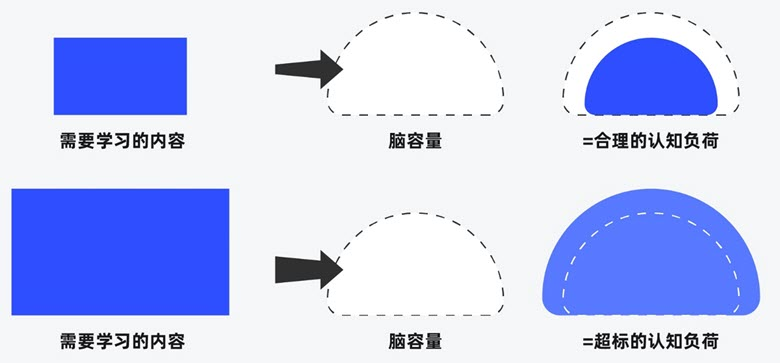##### 界面设计和游戏设计中的认知负荷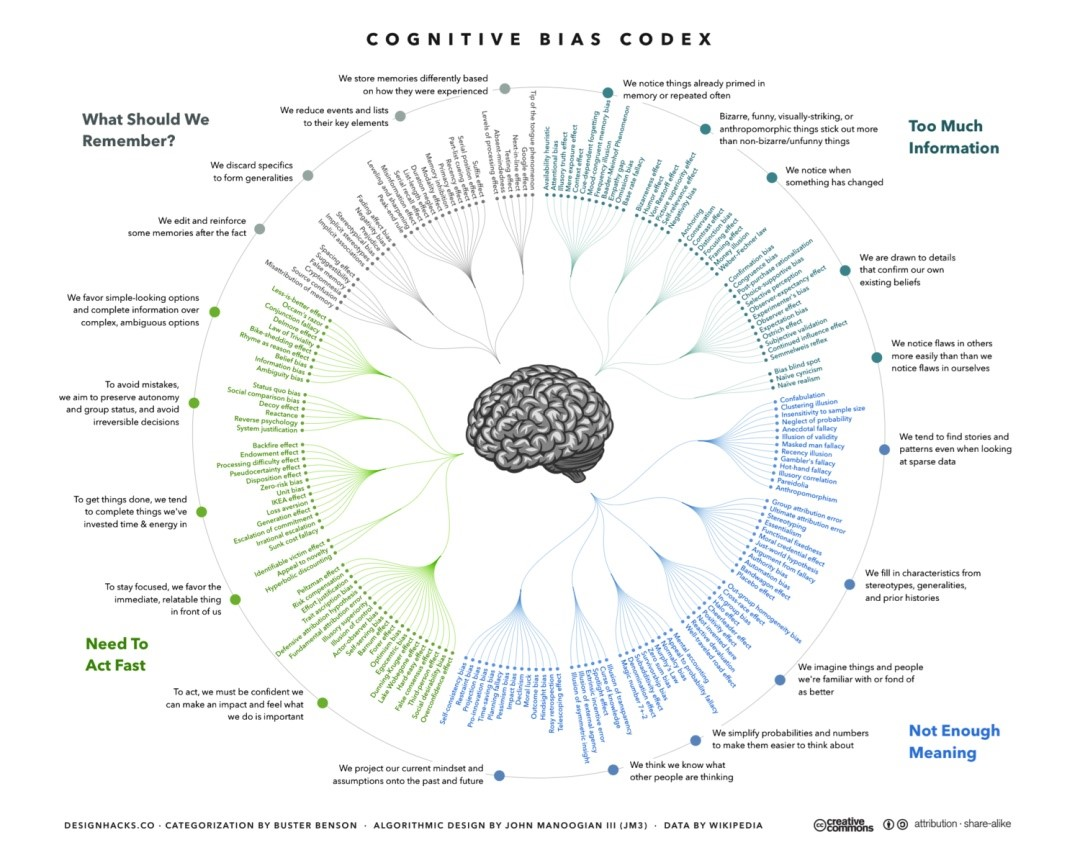##### 理解认知偏差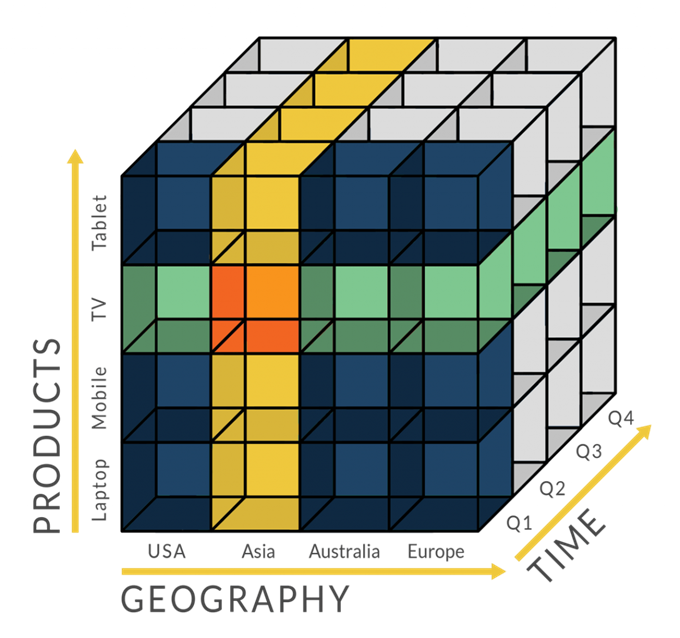## One Reply to “推荐算法之贝叶斯个性化排序 BPR”

1. hhmy说道：

请问博主，这篇文章的第一张插图的出处是哪本书？
标题是：Table 2.7：Characteristics of Pointwise Approach的插图